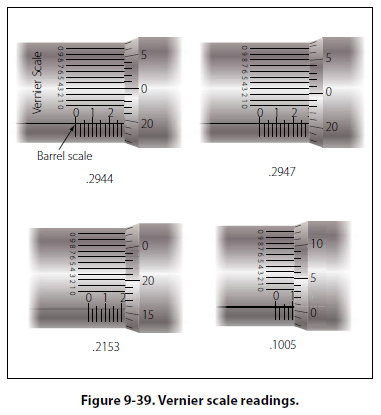Vernier Scale Some micrometers are equipped with a vernier scale that makes it possible to directly read the fraction of a division that is indicated on the thimble scale. Typical examples of the vernier scale as it applies to the micrometer are shown in Figure 9-39.All three scales on a micrometer are not fully visible without turning the micrometer, but the examples shown in Figure 9-38 are drawn as though the barrel and thimble of the micrometer were laid out flat so that all three scales can be seen at the same time. The barrel scale is the lower horizontal scale, the thimble scale is vertical on the right, and the long horizontal lines (0 through 9 and 0) make up the vernier scale. In reading a micrometer, an excellent way to remember the relative scale values is to remember that the 0.025 inch barrel scale graduations are established by the lead screw (40 threads per inch). Next, the thimble graduations divide the 0.025 inch into 25 parts, each equal to 0.001 inch. Then, the vernier graduations divide the 0.001 inch into 10 equal parts, each equal to 0.0001 inch. Remembering the values of the various scale graduations, the barrel scale reading is noted. The thimble scale reading is added to it; then the vernier scale reading is added to get the final reading. The vernier scale line to be read is always the one aligned exactly with any thimble graduation. In the first example in Figure 9-39, the barrel reads 0.275 inch and the thimble reads more than 0.019 inch. The number 1 graduation on the thimble is aligned exactly with the number 4 graduation on the vernier scale. Thus, the final reading is 0.2944 inch. In the second example in Figure 9-39, the barrel reads 0.275 inch, and the thimble reads more than 0.019 inch and less than 0.020 inch. On the vernier scale, the number 7 graduation coincides with a line on the thimble. This means that the thimble reading would be 0.0197 inch. Adding this to the barrel reading of 0.275 inch gives a total measurement of 0.2947 inch. The third and fourth examples in Figure 9-39 are additional readings that would require use of the vernier scale for accurate readings to ten-thousandths of an inch.
 ©AvStop Online Magazine                                                                                                                                                      Contact Us              Return To Books